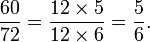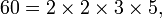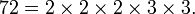# Greatest common divisor/Tutorial

(Main article: Greatest common divisor.)

## Explicit example

For example, the divisors of 60 are

1, 2, 3, 4, 5, 6, 10, 12, 15, 20, 30, 60,

and the divisors of 72 are

1, 2, 3, 4, 6, 8, 9, 12, 18, 24, 36, 72.

The common divisors of 60 and 72 are the numbers that appear in both lists:

1, 2, 3, 4, 6, 12.

The greatest common divisor of 60 and 72 is therefore 12. One writes "gcd(60, 72) = 12", or simply "(60, 72) = 12".

The greatest common divisor is used in reducing fractions to lowest terms, thus:$\frac{60}{72} = \frac{12 \times 5}{12 \times 6} = \frac{5}{6}.$

## An algorithm involving prime factorizations

One method of finding the greatest common divisor of two integers involves factoring both into prime numbers:$60 = 2 \times 2 \times 3 \times 5,\,$$72 = 2 \times 2 \times 2 \times 3 \times 3.\,$

Observe that the prime number 2 occurs twice in the factorization of 60, and three times in the factorization of 72. One says that the multiplicity of that prime factor is 2 in the first case and 3 in the second. ("Multiplicity" means the number of times the prime factor appears in the factorization.) For each prime factor, one sees which multiplicity is smaller: the one in the factorization of 60 or the one in the factorization of 72:

In the factorization of 60, the multiplicity of the prime factor 2 is 2.
In the factorization of 72, the multiplicity of the prime factor 2 is 3.
The smaller of those multiplicities is 2.

Next:

In the factorization of 60, the multiplicity of the prime factor 3 is 1.
In the factorization of 72, the multiplicity of the prime factor 3 is 2.
The smaller of those multiplicities is 1.

Next:

In the factorization of 60, the multiplicity of the prime factor 5 is 1.
In the factorization of 72, the multiplicity of the prime factor 5 is 0.
The smaller of those multiplicities is 0.

For completeness, note that

all other prime numbers have multiplicity 0 in the factorization of both 60 and 72.
The smaller of those two multiplicities (both 0) is 0.

Therefore

the multiplicity of 2 in the factorization of the gcd is 2;
the multiplicity of 3 in the factorization of the gcd is 1;
the multiplicity of any other prime number in the factorization of the gcd is 0.

The gcd is therefore 2 × 2 × 3 = 12.

Here is another example: the problem is to find gcd(14280, 1638). We have

14280 = 2 × 2 × 2 × 3 × 5 × 7 × 17.
1638 = 2 × 3 × 3 × 7 × 13.

For the prime number 2, the multiplicities are 3 and 1; the smaller of those is 1.

For the prime number 3, the multiplicities are 1 and 2; the smaller of those is 1.

For the prime number 5, the multiplicities are 1 and 0; the smaller of those is 0.

For the prime number 7, the multiplicities are 1 and 1; the smaller of those is 1.

For the prime number 11, the multiplicities are 0 and 0; the smaller of those is 0.

For the prime number 13, the multiplicities are 0 and 1; the smaller of those is 0.

And so on—for all larger prime numbers the multiplicity is 0.

Therefore gcd(14280, 1638) = 2 × 3 × 7 = 42.

## An algorithm not involving prime numbers

When the prime factors of a number are large, the algorithm above may be inefficient. Euclid's algorithm does not involve prime factorizations and runs fast in such cases. It may be described as follows:

(1) Replace the larger of the two numbers with the remainder on division of the larger by the smaller.
(2) Repeat step (1) until one of the two numbers is 0.
(3) The one that is not 0 is the gcd.

For an example, let's find the greatest common divisor of the last pair of numbers we used above, (14280, 1638).

14280 / 1638 = 8 remainder 1176. because 1638 times 8 is 13104.
Now we take the new pair of numbers (1638, 1176).
1638 / 1176 = 1 remainder 462
Now we have (1176,462).
1176 / 462 = 2 remainder 252
Now we have (462, 252).
462 / 252 = 1 remainder 210.
Now we have (252,210).
252 / 210 = 1 remainder 42.
Now we have (210,42).
210 / 42 = 5 remainder 0.
Now we have (42,0).

Therefore gcd(14280,1638) = 42.Some content on this page may previously have appeared on Citizendium.